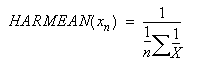﻿ HARMEAN | Spread Formula Reference
Formula Functions / Functions H to L / HARMEAN
In This Topic
HARMEAN
In This Topic

This function returns the harmonic mean of a data set.

#### Syntax

HARMEAN(value1,value2,...)

HARMEAN(array)

HARMEAN(array1,array2,...)

#### Arguments

You can specify a set of numeric values. You can also use a single array or a reference to an array instead of arguments separated by commas. If an array or reference argument contains text, logical values, or empty cells, the function ignores those values; however, the function includes in calculations cells with the value zero. This function can have up to 255 arguments.

Data should be provided so that the value arguments should be greater than zero.

#### Remarks

The harmonic mean is always less than the geometric mean, which is always less than the arithmetic mean

The equation for this function is:#### Data Types

Accepts numeric data for all arguments. Returns numeric data.

#### Examples

HARMEAN(F1:F9)

HARMEAN(R1C6:R9C6)

HARMEAN(35,31,47,51,37,31,58,39) gives the result 39.2384929823

#### Version Available

This function is available in product version 1.0 or later.